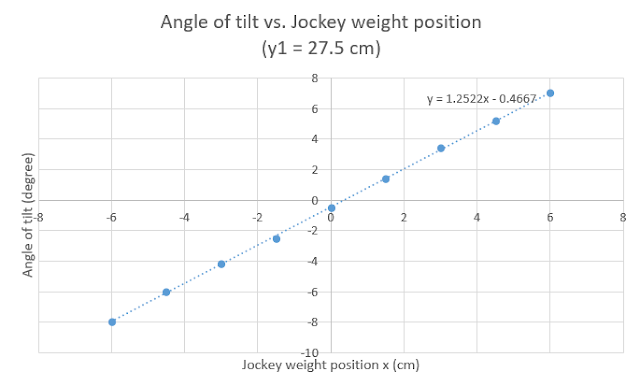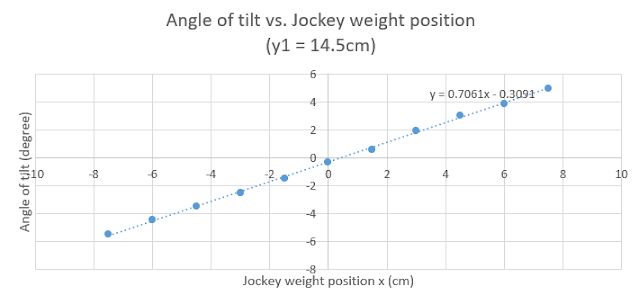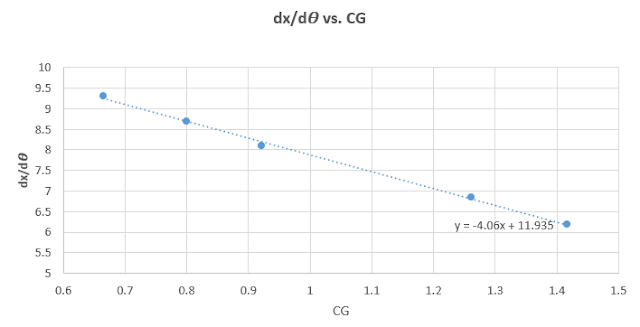### Abstract

The study of the meta-centric location and the stability of a floating body is very important in many applications such as designing of ships. The aim of this experiment is to study the forces acting on a floating body like the buoyant and gravity force which affect the stability of the body.

The experiment was done by locating the meta-centric heights and center of gravity position. The experiment was performed for different heights of the adjustable weight, and for different displacements of balancing weight, each time the height of the adjustable weight and the displacement of the jockey weight and the angle of tilt which changes due to changing the position of the balancing weight was recorded. In the end of this experiment, we predict to have an experimental value to be near to the theoretical value due to the specific procedures we worked on.Floating balloon

### Objectives

• Ø  Understand how the buoyant force acts on a floating body.
• Ø  Understand the effect of the position of the center of gravity on floating bodies.
• Ø  Calculate the height of G above the base (dx/dθ) for different heights of adjustable weight.
• Ø  Find the gradient stability line (Ῡ) for each run.
• Ø  Calculate CG, GM and CM.
• Ø  Sketch charts between the angle of tilt and jockey weight position.

### Data and calculations

Y1= 30.5cm
Ῡ= 9.3cm

Table-1: collected data

Position of jockey weight X1 (cm)

y1 (cm)

-7.5

-6

-4.5

-3

-1.5

0

1.5

3

4.5

6

7.5

30.5

-

-

-7.5

-5.2

-2.8

0

2.0

3.9

6.2

8.3

-

27.5

-

-8.0

-6.0

-4.2

-2.5

-0.5

1.4

3.4

5.2

7.0

-

24.5

-8.5

-7.0

-5.0

-3.5

-2.0

-0.5

1.1

3.0

4.5

6.0

8.0

18.0

-6.0

-5.0

-4.0

-2.5

-1.5

-0.4

0.8

2.0

3.5

4.5

5.8

14.5

-5.5

-4.5

-3.5

-2.5

-1.5

-0.3

0.6

1.9

3.0

3.9

5.0
Angle of tile (degrees)

### Calculations

1. To calculate the constant A

We calculate it using the initial condition at Ῡ= 9.3cm and y1= 30.5cm , from the following equation:

Where
: The height of G above base.
y: The height of adjustable weight above base.
A: Is a constant to be determined from the first measurement.

1)   2. To calculate the distance CM using the experimental data

Where:
: Jockey weight [0.2 kg]
W: Total weight of assembly [2.6 kg]
V: Total displacement volume = W/ = 2.6/1000 = 0.0026 [m3]
L: Length of pontoon [0.36 m]
D: Breadth of pontoon [0.206 m]

3. Finding the value of CMexp

### Results

Table-2: Calculations of CM

y1 (cm)

ỹ (cm)

A (cm)

dx/d𝛳 (mm/degree)

GM (cm)

CG (cm)

Experimental CM (cm)

30.5

9.30

3.4

0.6642

0.0511

9.295

9.346

27.5

8.69

3.4

0.7990

0.0615

8.685

8.747

24.5

8.11

3.4

0.9213

0.0709

8.105

8.176

18.0

6.86

3.4

1.2615

0.0970

6.855

6.952

14.5

6.19

3.4

1.4162

0.1089

6.185

6.294Graph No.1: The relation between the angles of the tilt vs. the jockey weight position when y1 = 30.5 cm.Graph No.2: The relation between the angles of the tilt vs. the jockey weight position when y1 = 27.5 cm.Graph No.3: The relation between the angles of the tilt vs. the jockey weight position when y1 = 24.5 cm.Graph No.4: The relation between the angles of the tilt vs. the jockey weight position when y1 = 18.0 cm.Graph No.5: The relation between the angles of the tilt vs. the jockey weight position when y1 = 14.5 cm.Graph No.6: Shows the relation between the dx/dθ vs. CG

### Discussion of results

It is clear from graphs No.(1-5) that there is a linear relationship between the angle of tilt and the position of the jockey weight, the graphs No.(1-5) also show a value of  when x=0, this is due to systematic errors in the device and blunder errors (human errors). It is noticed that each graph shows positive readings when the weight is moved to the right and negative readings when the weight is moved to the left, whereas each displacement has approximately the same tilt angle with minor differences due to the errors mentioned above.

The values of the change in position of jockey weight with respect to tilt angle (dx/dθ) were found for each graph No.(1-5) and were drawn versus the position of the center of gravity (CG) in graph No.6. It is noticed that there is an inverse relationship between (dx/dθ) and CG and as the ratio (dx/dθ) decreases the stability of the floating body will decrease.

### Conclusion

In this experiment the pontoon was floated in water for different positions of the adjustable weight, the jockey weight was moved from its central position to both sides in increments of 1.5 cm each time and the angle of tilt was measured for each step.

The relationship between the angle of tilt  in degrees and the position of the jockey weight (x) in mm was found to be a linear relationship, and the gradient of each line represents (dx/dθ). Another graph was plotted to represent the change in position of jockey weight with respect to tilt angle (dx/dθ) versus the position of the center of gravity (CG), and the relation between them was linear with negative slope.

The results of this experiment can be improved by improving the devices used and the conditions of the environment that the experiment was performed in, like using different shapes of the pontoon, or using more accurate one with perfect weight distribution, and using more accurate tilt needle. Some of the environmental conditions which could affect the results are vibrations and the slope in the lab floor. Another source of errors are blunder errors, like not placing the jockey weight in the right position or taking wrong reading of the height of the balancing weight, and taking the reading of the angle while not looking perpendicularly at the angle meter.